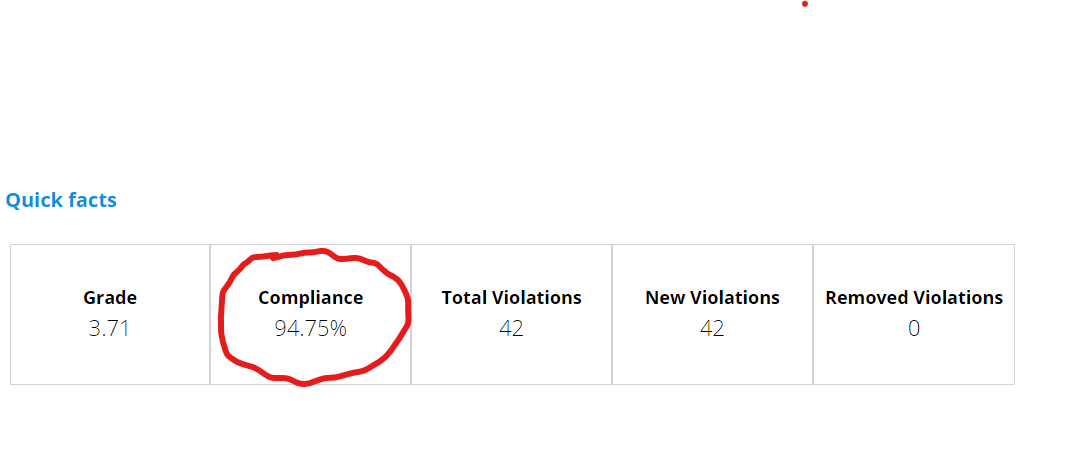### Post

1 follower Follow

# Calculation total "Compliance %" for CISQ, ISO5055

Hello everyone,
I have a problem in correctly calculating the total Compliance, see the attached screen as an example, which can be found in the table of the "Quick facts" within the CISQ rules, ISO5055.I apply the following formula:
(Total check- Failed check / Total check) * 100

But the result that returns is wrong compared to what is displayed in HD.

Can you tell me how I can correctly calculate the total Compliance?

Thank you